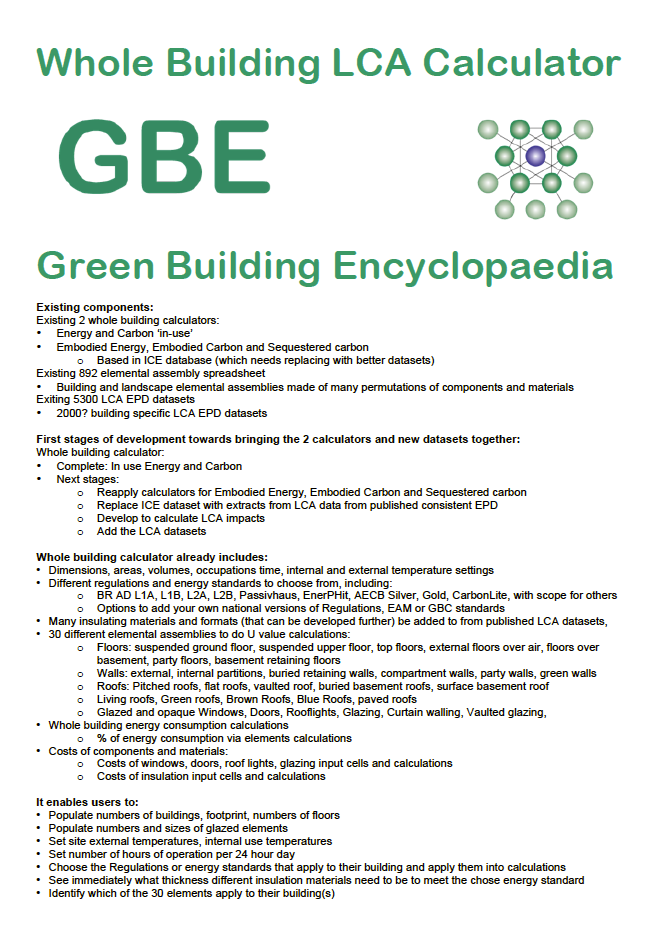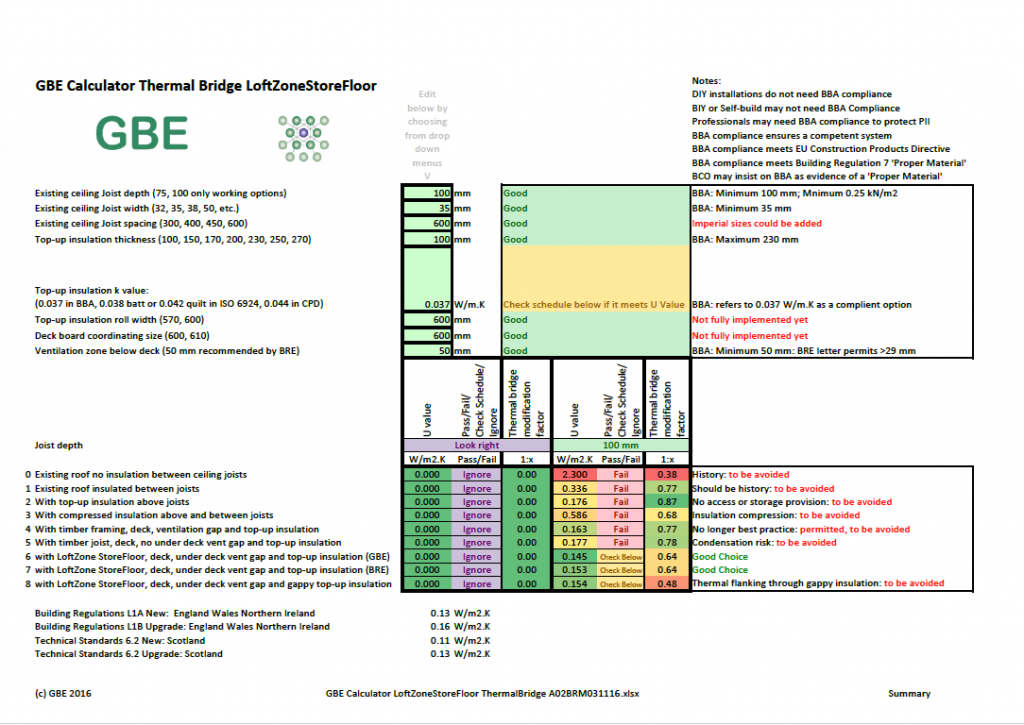Category

# Calculators

GBE Equations Navigation GBE > Encyclopaedia > Files > Calculators > Equations > G#31163 GBE Equations Navigation Index: GBE Equations Decrement Delay Factor (Equation) G#31148 U Value (Equation) G#_____ Psi...